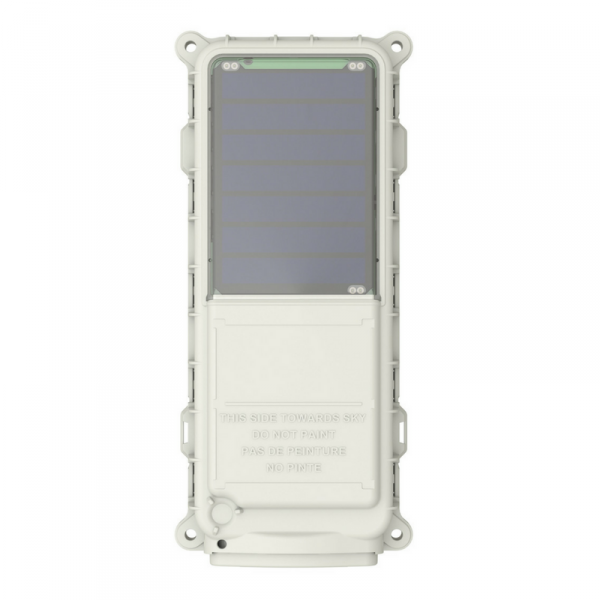# Globalstar SmartOne SolarПроизводитель: Globalstar
Обсуждение на форуме

Количество объектов

335
Онлайн
729
Всего

Для того чтобы система мониторинга Wialon корректно идентифицировала данные от Globalstar SmartOne Solar в диалоге настроек объекта, укажите следующие данные :

 Тип устройства: Globalstar SmartOne Solar Уникальный ID: custom

Укажите следующие параметры в конфигурационной программе или в конфигурации Globalstar SmartOne Solar для использования его в Wialon Hosting :

 Порт сервера: 21669

Всего в серверном центре Wialon используется 729 объектов Globalstar SmartOne Solar — это составляет 0.5% от общего количества устройств в категории «Стационарные устройства».

График изменения количества подключений в серверном центре Wialon за последние 30 дней:Имя параметра Описание Единицы измерения
input_status

These bits reflect the state of the inputs and indicate which one, if any, triggered an Input Status Changed message. Bit 0: Input 1 change: 0 = Did not trigger message, 1 = Triggered message. Bit 1: Input 1 state: 0 =
Closed, 1 = Open Bit 2: Input 2 change: 0 = Did not trigger message, 1 = Triggered message Bit 3: Input 2 state: 0 = Closed, 1 = Open.

msg_sub_type

Message sub-type code.

Value is 0 in the location message.

Value is 1 in the Device Turned On message.

Value is 2 in the Change of Loction Area alert message.

Value is 3 in the Input Status Changed message.

Value is 4 in the Undesired Input State message.

Value is 5 in the Re-Centering message.

Value is 6 in the Speed & Heading message

vibr

Value 1 = Unit is in a state of vibration. Value 0 = Unit is not in a state of vibration.

gps_fix_2d

Value 1 = GPS data reported is from a 2D fix. (Only 3 satellites were used in the fix.) Value 0 = GPS data reported is from a 3D fix.

motion

Value 1 = Device was In-Motion when the message was transmitted. Value 0 = Device was At-Rest when the message was transmitted.

num_tries

Number of Transmissions (1 to 15 Transmissions)

min_trans_interval

Minimum Interval between transmission attempts ( 5 second resolution) Default is 300 seconds

max_trans_interval

Maximum Interval between transmission attempts, Default is 600 seconds

gps_mean_search_time

Unsigned binary count in seconds for mean GPS search to acquire

gps_fails

Unsigned binary count of failed GPS attempts since last Diagnostic message

alarm Alarm Statistics
alarm1_count Alarm2 since last message (b7=0) / accum hours (b7=1)
alarm2_count

Alarm3 since last message (b7=0) / accum hours (b7=1)

transmissions Transmissions since last statistics message
input№_undesired_state_time

Accumulated time that Input 1 has been in its undesired state with a resolution of 10 minutes. For example: 1 hour would be represented as a value of 6, 2 hours as a value of 12, etc. This resolution results in a rollover rate of a total time accumulation of approximately 1.25 years. (OxFFFF means that Input 1 Accumulate has been turned off)

vibration_time

Accumulated time that the SMARTONE has been in a State of Vibration with a resolution of 10 minutes. For example: 1 hour would be represented as a value of 6, 2 hours as a value of 12, etc. This resolution results in a rollover rate of a total time accumulation of approximately 1.25 years. (OxFFFF means that Input 1 Accumulate has been turned off)

input№_switches_number

The total number of openings or closings of input 1 as selected by the user. (OxFF means that Input 1 Accumulate has been turned off)

transms_num

Number of transmissions per burst.

bat

Battery Condition. 0 = Good battery. 1 = Replace Battery

gps

GPS Subsystem Fault. 0 = GPS system OK. 1 = Fault.

transmt

Transmitter Subsystem Fault. 0 = Transmitter OK. 1 = Fault.

sched

Scheduler Subsystem Fault. 0 = OK. 1 = Fault.

msg_type

0 Standard Message Type
3 Non Standard message type

vibr_trigger

Value 1 = This message is being sent because

Transmit on Change of Vibration State is selected and the

vibration just changed state, or Undesired Vibration State

is selected and the vibration is in the undesired state.

Value 0 = This message is being transmitted for a reason

other than the above reasons.

input_state

These bits reflect the state of the Inputs and indicate

which one, if any, triggered an Input Status Changed

message.

Bit 0: Input 1 change: 0 = Did not trigger message, 1 =

Triggered message.

Bit 1: Input 1 state: 0 = Closed, 1 = Open

Bit 2: Input 2 change: 0 = Did not trigger message, 1 =

Triggered message

Bit 3: Input 2 state: 0 = Closed, 1 = Open

input1_undesired_state_time

Accumulated time that Input 1 has been in its undesired

state with a resolution of 10 minutes. For example: 1

hour would be represented as a value of 6, 2 hours as a

value of 12, etc. This resolution results in a rollover rate

of a total time accumulation of approximately 1.25 years.

(OxFFFF means that Input 1 Accumulate has been

turned off)

input1_switches_number

The total number of openings or closings of input 1 as

selected by the user. (OxFF means that Input 1

Accumulate has been turned off)

input2_undesired_state_time

Accumulated time that Input 2 has been in its undesired

state with a resolution of 10 minutes. For example: 1

hour would be represented as a value of 6, 2 hours as a

value of 12, etc. This resolution results in a rollover rate

of a total time accumulation of approximately 1.25 years.

(OxFFFF means that Input 2 Accumulate has been

turned off)

input2_switches_number

The total number of openings or closings of input 2 as

selected by the user. (OxFF means that Input 2

Accumulate has been turned off)

Шаг 1
Шаг 2
Шаг 3
Имя и фамилия*
Компания*
Email*
Телефон